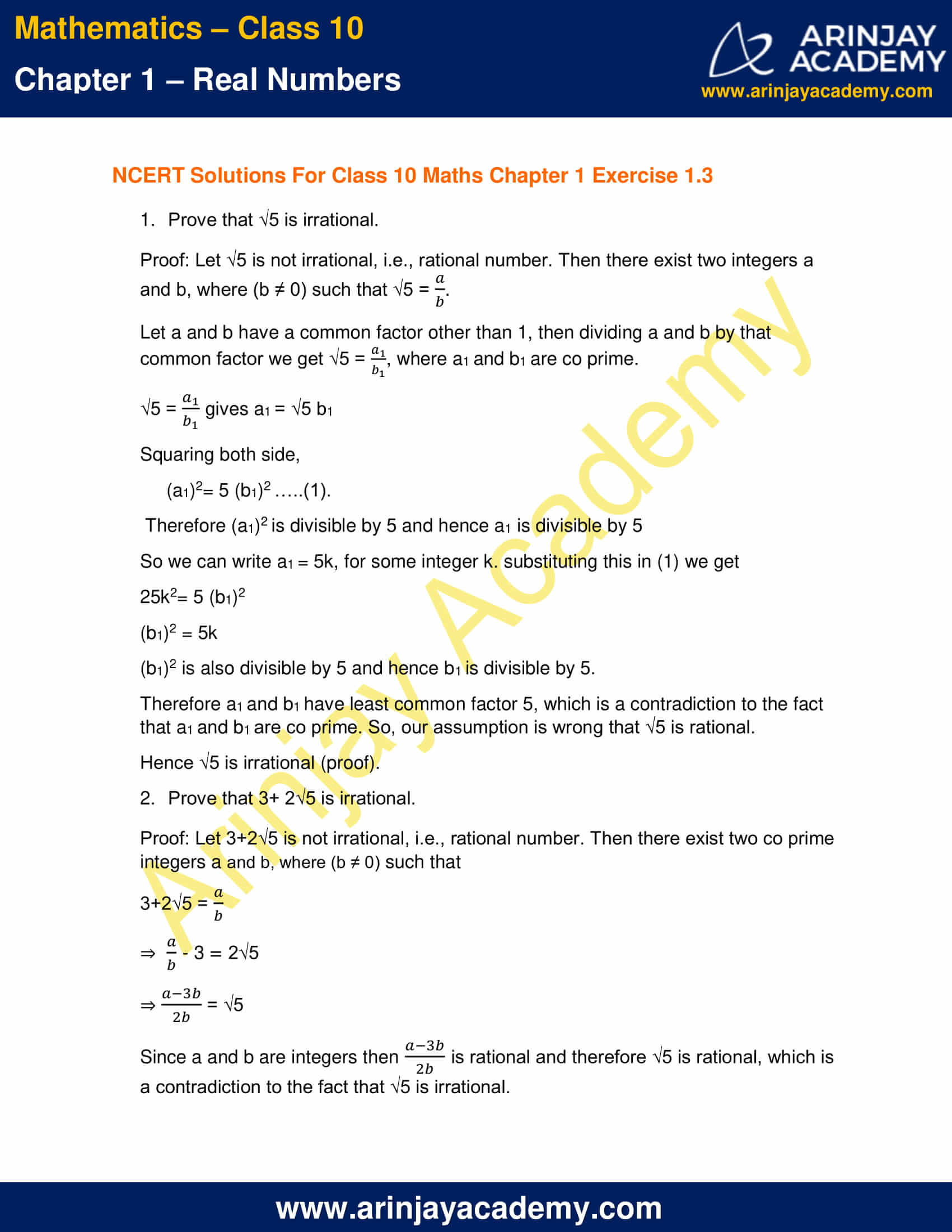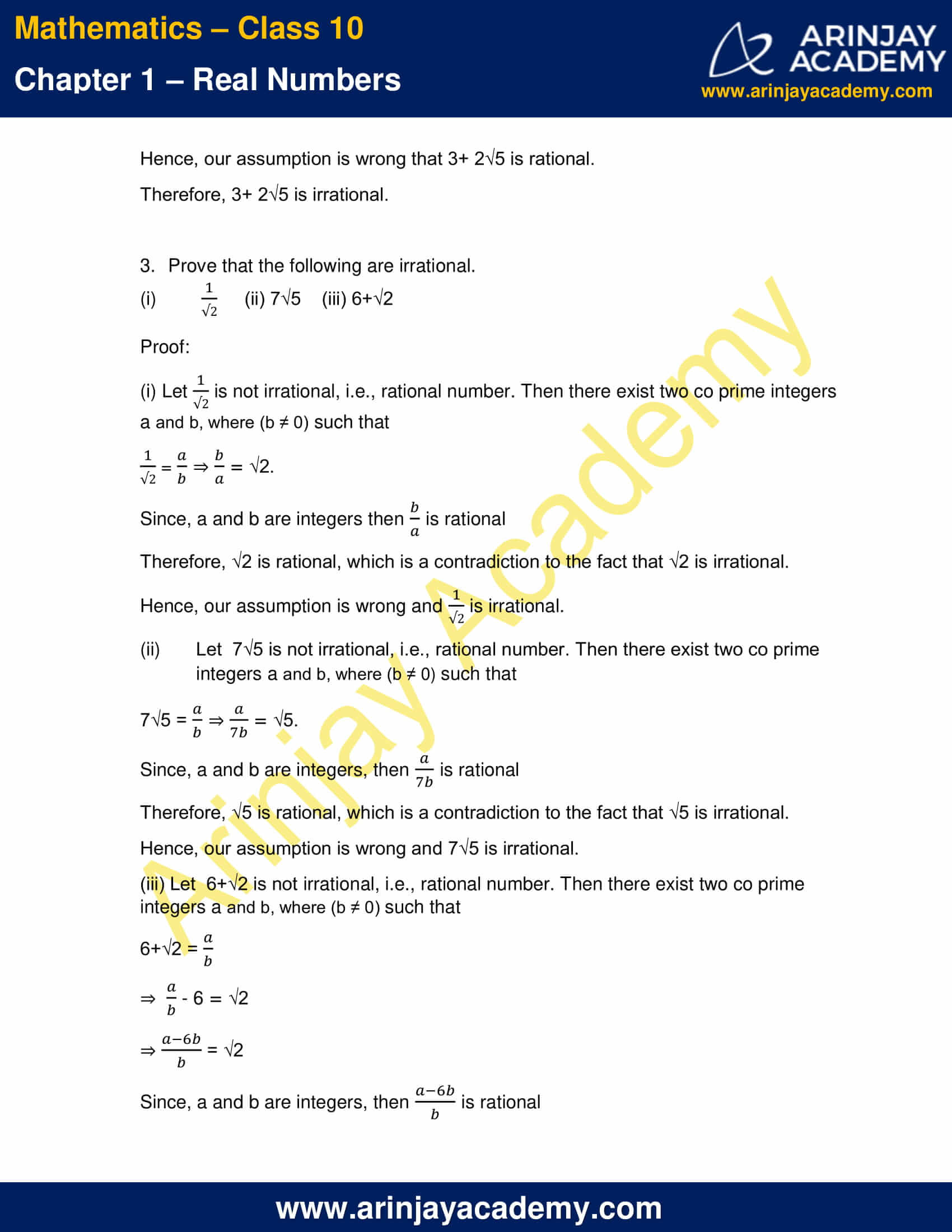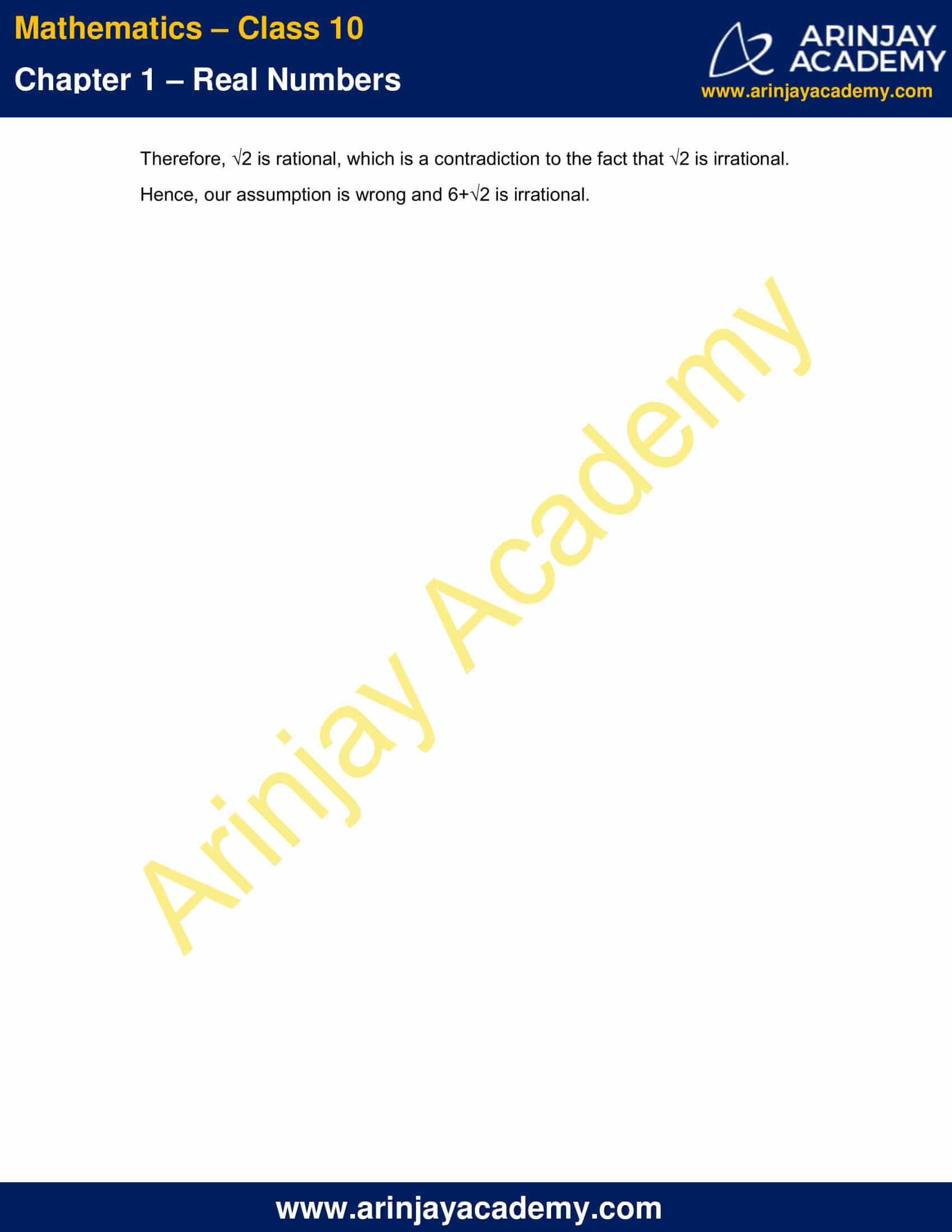# NCERT Solutions For Class 10 Maths Chapter 1 Exercise 1.3 – Real Numbers

Download NCERT Solutions For Class 10 Maths Chapter 1 Exercise 1.3 – Real Numbers. This Exercise contains 3 questions, for which detailed answers have been provided in this note. In case you are looking at studying the remaining Exercise for Class 10 for Maths NCERT solutions for other Chapters, you can click the link at the end of this Note.### NCERT Solutions For Class 10 Maths Chapter 1 Exercise 1.3 – Real NumbersNCERT Solutions For Class 10 Maths Chapter 1 Exercise 1.3 – Real Numbers

1. Prove that √5 is irrational.

Proof: Let √5 is not irrational, i.e., rational number. Then there exist two integers a and b, where (b ≠ 0) such that √5 = a/b

Let a and b have a common factor other than 1, then dividing a and b by that common factor we get √5 = a1/b1, where a1 and b1 are co prime.

√5 = a1/b1 gives a1 = √5 b1

Squaring both side,

(a1)2= 5 (b1)2 …..(1).

Therefore (a1)2 is divisible by 5 and hence a1 is divisible by 5

So we can write a1 = 5k, for some integer k. substituting this in (1) we get

25k2= 5 (b1)2

(b1)2 = 5k

(b1)2 is also divisible by 5 and hence b1 is divisible by 5.

Therefore a1 and b1 have least common factor 5, which is a contradiction to the fact that a1 and b1 are co prime. So, our assumption is wrong that √5 is rational.

2. Prove that 3+ 2√5 is irrational.

Proof: Let 3+2√5 is not irrational, i.e., rational number. Then there exist two co prime integers a and b, where (b ≠ 0) such that

3+2√5 = a/b

⇒ a/b – 3 = 2√5

⇒ (a-3b)/2b = √5

Since a and b are integers then (a-3b)/2b is rational and therefore √5 is rational, which is a contradiction to the fact that √5 is irrational.

Hence, our assumption is wrong that 3+ 2√5 is rational.

Therefore, 3+ 2√5 is irrational.

3. Prove that the following are irrational.

(i) 1/√2
(ii) 7√5
(iii) 6+√2

(i) Let 1/√2 is not irrational, i.e., rational number. Then there exist two co prime integers a and b, where (b ≠ 0) such that

1/√2 = a/b

⇒ b/a  = √2.

Since, a and b are integers then b/a  is rational

Therefore, √2 is rational, which is a contradiction to the fact that √2 is irrational.

Hence, our assumption is wrong and 1/√2 is irrational.

(ii) Let 7√5 is not irrational, i.e., rational number. Then there exist two co prime integers a and b, where (b ≠ 0) such that

7√5 = a/b

⇒ a/7b = √5.

Since, a and b are integers, then a/7b is rational

Therefore, √5 is rational, which is a contradiction to the fact that √5 is irrational.

Hence, our assumption is wrong and 7√5 is irrational.

(iii) Let  6+√2 is not irrational, i.e., rational number. Then there exist two co prime integers a and b, where (b ≠ 0) such that

6+√2 = a/b

⇒ a/b  – 6 = √2

⇒ (a – 6b/b) = √2

Since, a and b are integers, then (a – 6b/b) is rational

Therefore, √2 is rational, which is a contradiction to the fact that √2 is irrational.

Hence, our assumption is wrong and 6+√2 is irrational.

NCERT Solutions for Class 10 Maths Chapter 1 Exercise 1.3 – Real Numbers, has been designed by the NCERT to test the knowledge of the student on the topic – Revisiting Irrational Numbers